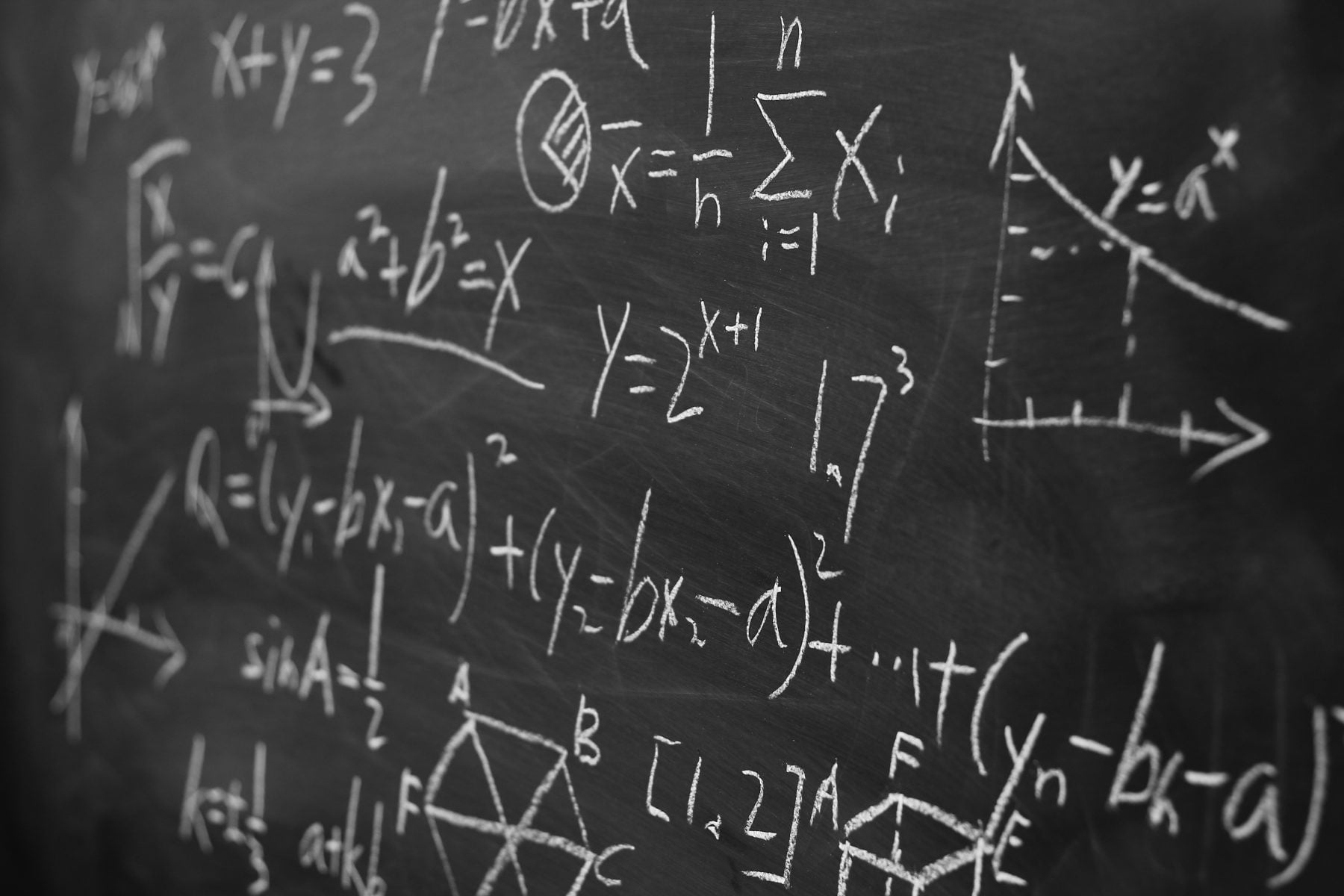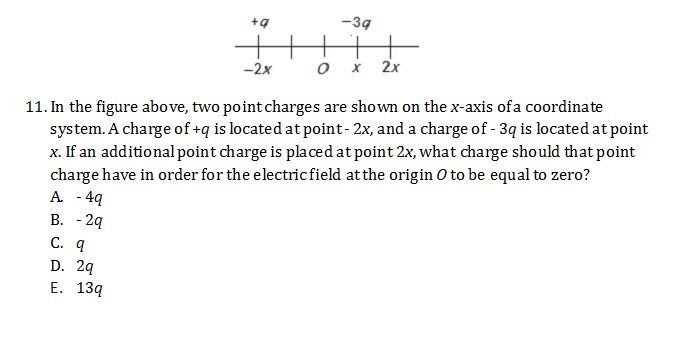# The SAT Physics Subject TestBy Lisa Mayo

Let's take a look at the SAT Physics Subject Test.

#### What does the Physics Subject Test Look Like?

The Physics Subject Test is a one-hour long, 75-question, multiple choice exam that covers mechanics, electricity and magnetism, waves, optics, thermodynamics, modern physics, and a handful of miscellaneous concepts such as chaos theory. It’s important to note that you’re not allowed to use a calculator on the exam. Fortunately however, test writers know this and create their exams accordingly, so you’re not likely to see questions that involve lengthy calculations. You also won’t see any calculus on the exam.

What types of questions will you see on the SAT Physics Subject Test? Check out the sample question below.

Example:#### How is the Exam Scored?

For each correct answer, you’ll earn 1 point, and for each incorrect answer, you’ll lose  point. If you leave a question blank, you’ll receive 0 points for that question. Your total points for the test will be added to form a raw score, then that raw score will be converted to a scaled score that can range from 200–800. The average scaled score on the Physics Subject Test is approximately 690, but fortunately the test has a very generous curve. On most tests, you can leave as many as 15 questions blank and still score an 800, assuming that you answer all of the remaining questions correctly.

#### What Skills from School Will Help Me on the Test?

If you’ve taken a full year of physics, you’ll find that you can put most of your physics knowledge to use on the exam. The equations you’ve come to know and love so well in physics class will show up on the test, and since you won’t get a formula sheet, knowing your formulas is vital!

You’ll also have an opportunity to put your math skills to use on the Physics Subject Test; again, you won’t need calculus, but a good understanding of trigonometry and scientific notation will definitely come in handy, as will an ability to estimate when calculating.

#### Are There Any Specific Classes I Can Take that Will Help Me Prepare?

While not required, taking an AP (Advanced Placement) or IB (International Baccalaureate) physics class may be helpful. In particular, most AP Physics 2 and IB Physics HL classes cover the majority of the content on the exam.

Most AP and IB classes are more focused on academic content than they are on test-taking strategies. For example, students will learn relevant concepts and formulas, but they likely won’t learn how to spot wrong answer choices, use the time to their advantage, or minimize the guessing penalty. To learn how to game the test, consider working with a prep book, study program, or tutor who specializes in the Physics Subject Test.

Find out exactly how much strategy and content from your current AP or IB courses can be applied to the SAT Physics Subject Test! Download our handy guide here.

#### Are There Any Tricks I Can Use to Immediately Improve My Score?

Write down formulas! If a question references the electric field, write down the formula for the electric field and put in what you know from the question. If a question mentions torque, write down the formula for torque and put in what you know. Even if you’re initially not entirely sure how you can use the formula, you’ll often find that once you start putting in what you know, you can solve the problem.

Also, focus on estimating rather than on calculating. In physics class, you need specific values: g = 9.81 m/s2π = 3.14, and so forth. Your grade may even depend upon the level of precision that you give to your numbers. On the SAT Physics Subject Test however, you won’t need answers that are so precise; you’ll only need answers that are close enough to the actual values to allow you to choose one of five answer choices. If you say g is 10 m/s2­ and π is approximately 3, for example, you’ll be able to identify the correct answer and also save yourself some complicated calculations.

#### I'm an International Student. Any Tips for Me?

Fortunately, even if you’ve taken physics in a country other than the U.S., the concepts tested on the SAT Physics Subject Test are likely to be ones you’ve seen in your own classes. However, the wording on the exam is designed to be a bit tricky, so reading carefully is important. Take long questions in bite-sized pieces, focusing on one question at a time and calculating as you go.

Main Takeaways:

• The concepts tested on the SAT Physics Subject Test include mechanics, electricity and magnetism, waves, optics, thermodynamics, modern physics, and a few miscellaneous topics that vary by test.
• The average score on this exam is about a 690 (out of 800).
• Knowing your formulas is critical! Start creating flashcards now, and, once you have your formulas memorized, be sure to write them down and use them while you’re taking the actual test.
• When possible, estimate; don’t calculate specific values unless you can’t identify the correct answer otherwise.
• If you’re an international student who took physics in a language other than English, be sure to work long problems in bite-sized pieces.
##### --